Next: Bayesian Inference Up: Data Mining Previous: Decomposition

# Bayesian Networks

Bayesian networks is a statistical method for Data Mining, a statistical method for discovering valid, novel and potentially useful patterns in data. A description of Probabilistic networks and machine learning techniques is given in .

Bayesian networks are used to represent essential information in databases in a network structure. The network consists of edges and vertices, where the vertices are events and the edges relations between events. A simple Bayesian network is illustrated in Fig 3.2, where symptoms are dependent on a disease, and a disease is dependent on age, work and work environment. Introductions to Bayesian networks can be found in , , ,  and .

Bayesian networks are easy to interpret for humans, and are able to store causal relationships , that is, relations between causes and effects. The networks can be used to represent domain knowledge, and it is possible to control inference and produce explanations on a network.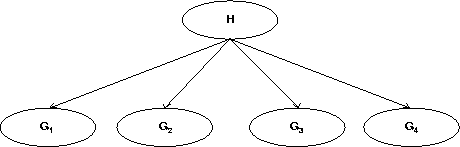A simple usage of Bayesian networks is denoted naive Bayesian classification . These networks consist only of one parent and several child nodes as in Fig 3.3. Classification is done by considering the parent node to be a hidden variable (H in the figure) stating which class (child node) each object in the database should belong to. An existing system using naive Bayesian classification is AutoClass .

The theoretical foundation for Bayesian networks is Bayes rule, which states: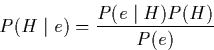Where H is a hypothesis, and e an event.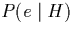is the posterior probability, and P(H) is the prior probability. A proof of Bayes rule is given in appendix A.

To give a formal definition of Bayesian networks, we introduce some terminology which is taken from :

If a subset of Z nodes in a graph G intercepts all paths between the nodes X and Y (written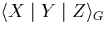), then this corresponds to conditional independence between X and Y given Z: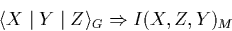conversely: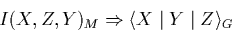with respect to some dependency model M.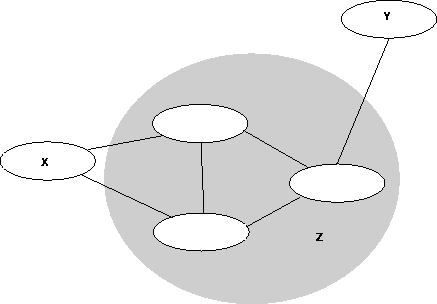A Directed, Acyclic Graph (DAG) D is said to be a I-map of a dependency model M if for every three disjoint sets of vertices, X, Y and Z we have:A DAG is a minimal I-map of M if none of its arrows can be deleted without destroying its I-mapness.

Given a probability distribution P on a set of variables U, a DAG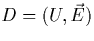is called a Bayesian Network of P iffD is a minimal I-map of P.

A Bayesian network is shown in Fig 3.3, representing the probability distribution P: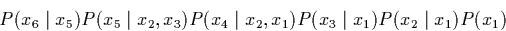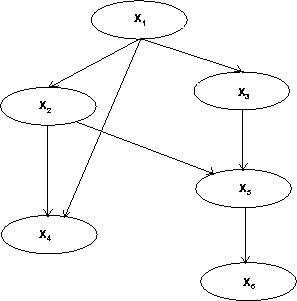Next: Bayesian Inference Up: Data Mining Previous: Decomposition
Torgeir Dingsoyr
2/26/1998